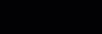cancel
Showing results for
Did you mean:New Member

## Average of 3 weeks without using time intellige functions

Hi there,

I'm trying to create a kind rolling average over the last 3 weeks but without using time intelligent functions.

My excel looks like this:

 A B C D E F G 1 WEEK 1 WEEK 2 WEEK 3 WEEK 4 WEEK 5 WEEK 6 2 Observation / Total Items 10% 15% 5% 2% 8% 10% 3 Last 3 Week average 10% 7% 5% 7%

And the excel formula from D3 woud be =AVERAGE(B2:D2),  E3 =AVERAGE(C2:E2),  F3=AVERAGE(D2:F2), and G3=AVERAGE(E2:G2)

What would be the best way to do it in Power Bi?

My objetive is to use a "Line and clustered column chart" where my week is on X-Axis, my total Items as a Column y-axis and my Observations/Items as a Line y-axis

Just in case my data looks like this:

 Table 1 Date Week 1/1/2021 1 4/1/2021 1 7/1/2021 1 10/1/2021 2 13/1/2021 2 16/1/2021 2 19/1/2021 3 22/1/2021 3

(My tables are already related by date column)

 Table 2 Date Items Observations 1/1/2021 72 66 4/1/2021 99 37 7/1/2021 89 18 10/1/2021 81 30 13/1/2021 84 12 16/1/2021 70 30 19/1/2021 77 6 22/1/2021 84 76

by the way, I'm using radom data just to give an example of what the data looks like.

Any suggestion would be greate...

1 ACCEPTED SOLUTIONSolution Sage

If I understand correctly, you can create a table of averages

Averages Table =
SUMMARIZE(dimDate,dimDate[Week],"_averages",DIVIDE(SUM(factItems[Observations]),SUM(factItems[Items])))
and then create a measure from that table
Rolling 3 Week Average =
var _currentWeek =
SELECTEDVALUE(dimDate[Week])
var _firstWeek =
_currentWeek - 2
Return
IF(
_firstWeek > 0,
CALCULATE(
AVERAGE('Averages Table'[_averages]),
FILTER(all('Averages Table'), 'Averages Table'[Week] >= _firstWeek && 'Averages Table'[Week] <= _currentWeek)
)
)
6 REPLIES 6Solution Sage

If I understand correctly, you can create a table of averages

Averages Table =
SUMMARIZE(dimDate,dimDate[Week],"_averages",DIVIDE(SUM(factItems[Observations]),SUM(factItems[Items])))
and then create a measure from that table
Rolling 3 Week Average =
var _currentWeek =
SELECTEDVALUE(dimDate[Week])
var _firstWeek =
_currentWeek - 2
Return
IF(
_firstWeek > 0,
CALCULATE(
AVERAGE('Averages Table'[_averages]),
FILTER(all('Averages Table'), 'Averages Table'[Week] >= _firstWeek && 'Averages Table'[Week] <= _currentWeek)
)
)New Member

It's pretty close.

What I really need is the chart that I mention before more than the summarize table, so I modify your formula a little bit, and looks like this:

Rolling 3 Week Average
=
var _currentWeek =
SELECTEDVALUE(Table1[Week])
var _firstWeek =
_currentWeek - 2
Return
IF(
_firstWeek >= 0,
CALCULATE(
Calculos[% Observations/ Registers],
FILTER(all(Table1), Table1[Week] >= _firstWeek && Table1[Week] <= _currentWeek)
)
)

I did change _firstWeek >0 to _firstWeek >= 0

By the way, about Calculos[% Observations/ Registers]
Calculos is the table name (Spanish word of Calculations) and it is compose by the next measure:
Measure: % Observations/ Registers = DIVIDE([Items],[Observations],0)

My current data is
WEEK 0 = 100,00%
WEEK 1 =     5,33%
WEEK 2 =     4,19%
WEEK 3 =     7,80%

The average value for week 2 (week 0 to 2) is = 36,61%
And The average value for week 3 (week 1 to 3) is = 5,77%

But what I'm getting the following results with your formula:
WEEK 2 = 46,94%
WEEK 3 = 5,64%

I don't know why this difference is generated, maybe it is because I am using Calculos[% Observations/ Registers] as an expression, not sure at all.

Thanks for helping me...Solution Sage

It looks like you put the Calculos in the wrong table.

Try,

Make a new table with:

Calculos Table =
SUMMARIZE(Table1,Table1[Week],"_averages",[% Observations/ Registers])
Then make the following measure:
Rolling 3 Week Average =
var _currentWeek =
SELECTEDVALUE(Table1[Week])
var _firstWeek =
_currentWeek - 2
Return
IF(
_firstWeek >= 0,
CALCULATE(
AVERAGE('Calculos Table'[_averages]),
FILTER(all('Calculos Table'), 'Calculos Table'[Week] >= _firstWeek && 'Calculos Table'[Week] <= _currentWeek)
)
)
You need the first table created in order to take the average of the 3 weeks in the measure.New Member

You are right...

Thanks for the help. I'm really gladNew Member

What I'm looking for is a DAX for the step 2.

Stept 1: Formula where I can calculate average of my observations over Items by week from my table 2 (Which I already have).

Stept 2: Then I need to calculate the average from the 3 last weeks for each week (Like the first excel table example).

I already have the next formula:

Measure 1: Observation/ Items =
DIVIDE(Table2[Observations],Table2[Items],0)

Measure 2: % rolling average = AVERAGEX(FILTER(ALLSELECTED(Table1[Date]),Table1[Date]<=MAX(Table1[Date])), [ Observation/ Items])

My measure 2 It's an example of what I don't need but it's as far as I've come because it's a rolling average by day (this is what I mean of time intellingence functions, as far as I know it is a time intellingece function result). If I'm wrong about this measure, would be nice if you could correct me.

Hope this helps..Super User

@mzunino001 your question is not very clear, what do you mean by without time intelligence function also you want to use the last 3 weeks (what do you think week means here, is this not related to time? )

## ✨ Follow us on LinkedIn andto our YouTube channel

I would  Kudos if my solution helped. 👉 If you can spend time posting the question, you can also make efforts to give Kudos to whoever helped to solve your problem. It is a token of appreciation!

Subscribe to the @PowerBIHowTo YT channel for an upcoming video on List and Record functions in Power Query!!

Learn Power BI and Fabric - subscribe to our YT channel - Click here: @PowerBIHowTo

If my solution proved useful, I'd be delighted to receive Kudos. When you put effort into asking a question, it's equally thoughtful to acknowledge and give Kudos to the individual who helped you solve the problem. It's a small gesture that shows appreciation and encouragement! ❤

Did I answer your question? Mark my post as a solution. Proud to be a Super User! Appreciate your Kudos 🙂
Feel free to email me with any of your BI needs.